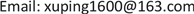﻿ 增强超立方体剖分图和全图的基尔霍夫指标 Kirchhoff Index in Subdivision and Total Graphs of Enhanced Hypercube

Vol. 08  No. 04 ( 2019 ), Article ID: 29868 , 6 pages
10.12677/AAM.2019.84078

Kirchhoff Index in Subdivision and Total Graphs of Enhanced Hypercube

Ping Xu

College of Mathematics and System Science, Xinjiang University, Urumqi XinjiangReceived: Apr. 2nd, 2019; accepted: Apr. 17th, 2019; published: Apr. 24th, 2019ABSTRACT

The resistance distance between any two vertices of G is defined as the network effective resistance between them if each edge of G is replaced by a unit resistor. The Kirchhoff index $Kf\left(G\right)$ is the sum of the resistance distances between all the pairs of vertices in G. In this paper, we obtained the relationship of Kirchhoff index between enhanced hypercube networks ${Q}_{n,k}$ and its two variant networks $s\left({Q}_{n,k}\right)$ and $t\left({Q}_{n,k}\right)$ , by deducing the characteristic polynomial of the Laplacian matrix related networks. Meanwhile, the special formulas for the Kirchhoff indexes of $s\left({Q}_{n,k}\right)$ and $t\left({Q}_{n,k}\right)$ were proposed, respectively.

Keywords:Enhanced Hypercube, Kirchhoff Index, Resistance Distance1. 引言

$G=\left(V,E\right)$ 中电阻距离的概念最早由Klein和Randić在  中提出来。图G是连通图， $V=\left\{{v}_{1},{v}_{2},\cdots ,{v}_{n}\right\}$$E=\left\{{e}_{1},{e}_{2},\cdots ,{e}_{m}\right\}$ 分别是图G的顶点集和边集。在图G中顶点vi和vj之间的电阻距离，记作 ${r}_{ij}$，指的是把图G中的每条边看作一个单位电阻由欧姆定律计算得到的节点vi和vj之间的有效电阻。图G中顶点vi和vj之间的普通距离，记作 ${d}_{ij}$，指的是vi和vj之间最短路的长度。在  中，图G的Wiener指标被定义为 $W\left(G\right)={\sum }_{i。在  中定义了一个与Wiener指标相似的和式 $Kf\left(G\right)={\sum }_{i，后来在  中被称作图G的基尔霍夫指标。Klein和Randić在  中证明了 ${r}_{ij}\le {d}_{ij}$，因此 $Kf\left(G\right)\le W\left(G\right)$，等式成立当且仅当图G是树；基尔霍夫指标在物理解释、电路学、图论、化学等方面有广泛的应用  。例如，Gutman和Mohar在  中已经证明有 $n\left(n\ge 2\right)$ 个顶点的连通图的基尔霍夫指标是它的顶点数与所有非零拉普拉斯特征值倒数之和的乘积。

2. 基本定义和引理

$Kf\left(G\right)=n\underset{i=1}{\overset{n-1}{\sum }}\frac{1}{{\mu }_{i}}$

1) ${P}_{L\left(l\left(G\right)\right)}={\left(x-2d\right)}^{m-n}{P}_{L\left(G\right)}\left(x\right)$

2) ${P}_{L\left(s\left(G\right)\right)}={\left(-1\right)}^{n}{\left(2-x\right)}^{m-n}{P}_{L\left(G\right)}\left(x\left(d+2-x\right)\right)$

3) ${P}_{L\left(t\left(G\right)\right)}=x\left(x-d-2\right){\left(x-2d-2\right)}^{m-n}\underset{i=1}{\overset{n-1}{\prod }}\left[\left({x}^{2}-2x-dx\right)+\left(3-2x+d\right){\mu }_{i}+{\mu }_{i}^{2}\right]$

$\frac{Kf\left({Q}_{n,k}\right)}{{2}^{n}}=-\frac{{a}_{{2}^{n}-2}}{{a}_{{2}^{n}-1}}$

$Kf\left({Q}_{n,k}\right)=\left\{\begin{array}{l}{2}^{n-1}\underset{t=0}{\overset{n}{\sum }}\underset{j=0}{\overset{n}{\sum }}\frac{1}{t+1}\left(\begin{array}{c}n-k+2\\ 2j\end{array}\right)\left(\begin{array}{c}k-1\\ t+1-2j\end{array}\right)\text{\hspace{0.17em}}\text{\hspace{0.17em}}\text{\hspace{0.17em}}\text{\hspace{0.17em}}\text{\hspace{0.17em}}n\equiv k\left(\mathrm{mod}2\right)\\ {2}^{n-1}\underset{t=0}{\overset{n-1}{\sum }}\underset{j=0}{\overset{n}{\sum }}\frac{1}{t+1}\left(\begin{array}{c}n-k+2\\ 2j\end{array}\right)\left(\begin{array}{c}k-1\\ t+1-2j\end{array}\right)\text{\hspace{0.17em}}\text{\hspace{0.17em}}\text{\hspace{0.17em}}\text{\hspace{0.17em}}\text{\hspace{0.17em}}n\overline{)\equiv }k\left(\mathrm{mod}2\right)\end{array}$

${\text{Spec}}_{L}\left({Q}_{n,k}\right)=\left\{0,{\left[2\right]}^{k-1},{\left[2t+2\right]}^{{\gamma }_{t}},{\left[2n+2\right]}^{{\gamma }_{n}}|t=1,2,\cdots ,n-1\right\}$

3. 增强超立方体剖分图和全图的基尔霍夫指标

1) $Kf\left(s\left({Q}_{n,k}\right)\right)={\left(n+3\right)}^{2}{2}^{n-2}\left(\underset{t=0}{\overset{n}{\sum }}\underset{j=0}{\overset{n}{\sum }}\frac{1}{t+1}\left(\begin{array}{c}n-k+2\\ 2j\end{array}\right)\left(\begin{array}{c}k-1\\ t+1-2j\end{array}\right)\right)+\left(n-1\right)\left(n+3\right){2}^{2n-3}+{2}^{n-1}$，若n和k有相同的奇偶性；

2) $Kf\left(s\left({Q}_{n,k}\right)\right)={\left(n+3\right)}^{2}{2}^{n-2}\left(\underset{t=0}{\overset{n-1}{\sum }}\underset{j=0}{\overset{n}{\sum }}\frac{1}{t+1}\left(\begin{array}{c}n-k+2\\ 2j\end{array}\right)\left(\begin{array}{c}k-1\\ t+1-2j\end{array}\right)\right)+\left(n-1\right)\left(n+3\right){2}^{2n-3}+{2}^{n-1}$，若n和k有不同的奇偶性。

$\begin{array}{c}{P}_{L\left(s\left({Q}_{n,k}\right)\right)}={\left(-1\right)}^{p}{\left(2-x\right)}^{q-p}{P}_{L\left({Q}_{n,k}\right)}\left(x\left(n+3-x\right)\right)\\ ={\left(-1\right)}^{p}{\left(2-x\right)}^{q-p}\left[{x}^{{2}^{n}}{\left(n+3-x\right)}^{{2}^{n}}+{a}_{1}{x}^{{2}^{n}-1}{\left(n+3-x\right)}^{{2}^{n}-1}+\cdots \\ \text{\hspace{0.17em}}\text{\hspace{0.17em}}+{a}_{{2}^{n}-2}{x}^{2}{\left(n+3-x\right)}^{2}+{a}_{{2}^{n}-1}x\left(n+3-x\right)\right]\end{array}$

${\left(-1\right)}^{p}{2}^{q-p}\left[{\left(n+3\right)}^{2}{a}_{{2}^{n}-2}-{a}_{{2}^{n}-1}-\left(q-p\right)\left(n+3\right){2}^{-1}{a}_{{2}^{n}-1}\right]$

$\begin{array}{c}\frac{Kf\left(s\left({Q}_{n,k}\right)\right)}{{2}^{n}+\left(n+1\right){2}^{n-1}}=-\frac{{\left(n+3\right)}^{2}{a}_{{2}^{n}-2}-{a}_{{2}^{n}-1}-\left(q-p\right)\left(n+3\right){2}^{-1}{a}_{{2}^{n}-1}}{\left(n+3\right){a}_{{2}^{n}-1}}\\ =-\left(n+3\right)\frac{{a}_{{2}^{n}-2}}{{a}_{{2}^{n}-1}}+\frac{q-p}{2}+\frac{1}{n+3}\\ =\frac{\left(n+3\right)}{{2}^{n}}Kf\left({Q}_{n,k}\right)+\frac{1}{n+3}+\left(n-1\right){2}^{n-2}\end{array}$

$Kf\left(s\left({Q}_{n,k}\right)\right)=\frac{{\left(n+3\right)}^{2}}{2}Kf\left({Q}_{n,k}\right)+\left(n-1\right)\left(n+3\right){2}^{2n-3}+{2}^{n-1}$

$Kf\left(s\left({Q}_{n,k}\right)\right)={\left(n+3\right)}^{2}{2}^{n-2}\left(\underset{t=0}{\overset{n}{\sum }}\underset{j=0}{\overset{n}{\sum }}\frac{1}{t+1}\left(\begin{array}{c}n-k+2\\ 2j\end{array}\right)\left(\begin{array}{c}k-1\\ t+1-2j\end{array}\right)\right)+\left(n-1\right)\left(n+3\right){2}^{2n-3}+{2}^{n-1}$

$Kf\left(s\left({Q}_{n,k}\right)\right)={\left(n+3\right)}^{2}{2}^{n-2}\left(\underset{t=0}{\overset{n-1}{\sum }}\underset{j=0}{\overset{n}{\sum }}\frac{1}{t+1}\left(\begin{array}{c}n-k+2\\ 2j\end{array}\right)\left(\begin{array}{c}k-1\\ t+1-2j\end{array}\right)\right)+\left(n-1\right)\left(n+3\right){2}^{2n-3}+{2}^{n-1}$

1) 若n和k有相同的奇偶性，则

$\begin{array}{c}Kf\left(t\left({Q}_{n,k}\right)\right)={2}^{n-1}+\frac{\left(n-1\right)\left(n+3\right)}{n+2}{2}^{2n-3}+\frac{\left(n+3\right)\left(3n+13\right)}{n+4}{2}^{n-2}\\ \text{\hspace{0.17em}}\text{\hspace{0.17em}}×\left(\underset{t=0}{\overset{n}{\sum }}\underset{j=0}{\overset{n}{\sum }}\frac{3t+n+7}{\left(t+1\right)\left(2t+n+6\right)}\left(\begin{array}{c}n-k+2\\ 2j\end{array}\right)\left(\begin{array}{c}k-1\\ t+1-2j\end{array}\right)\right)\end{array}$

2) 若n和k有不同的奇偶性，则

$\begin{array}{c}Kf\left(t\left({Q}_{n,k}\right)\right)={2}^{n-1}+\frac{\left(n-1\right)\left(n+3\right)}{n+2}{2}^{2n-3}+\frac{\left(n+3\right)\left(3n+13\right)}{n+4}{2}^{n-2}\\ \text{\hspace{0.17em}}\text{\hspace{0.17em}}×\left(\underset{t=0}{\overset{n-1}{\sum }}\underset{j=0}{\overset{n}{\sum }}\frac{3t+n+7}{\left(t+1\right)\left(2t+n+6\right)}\left(\begin{array}{c}n-k+2\\ 2j\end{array}\right)\left(\begin{array}{c}k-1\\ t+1-2j\end{array}\right)\right)\end{array}$

$\begin{array}{c}{\left(-1\right)}^{q-p}b={\left(2n+4\right)}^{q-p}\underset{i=1}{\overset{{2}^{n}-1}{\prod }}\left[{\mu }_{i}^{2}+\left(n+4\right){\mu }_{i}\right]+\left(n+3\right)\left(q-p\right){\left(2n+4\right)}^{q-p-1}\underset{i=1}{\overset{{2}^{n}-1}{\prod }}\left[{\mu }_{i}^{2}+\left(n+4\right){\mu }_{i}\right]\\ \text{\hspace{0.17em}}\text{\hspace{0.17em}}+\left(n+3\right){\left(2n+4\right)}^{q-p}\underset{j=1}{\overset{{2}^{n}-1}{\sum }}\left[\left(2+2{\mu }_{j}+d\right)\underset{i=1,i\ne j}{\overset{{2}^{n}-1}{\prod }}\left({\mu }_{i}^{2}+\left(n+4\right){\mu }_{i}\right)\right]\end{array}$

$c={\left(-1\right)}^{q-p-1}\left(n+3\right){\left(2n+4\right)}^{q-p}\underset{i=1}{\overset{{2}^{n}-1}{\prod }}\left[{\mu }_{i}^{2}+\left(n+4\right){\mu }_{i}\right]$

$\begin{array}{c}\frac{Kf\left(t\left({Q}_{n,k}\right)\right)}{q+p}=-\frac{b}{c}=\frac{1}{n+3}+\frac{q-p}{2\left(n+2\right)}+\underset{i=1}{\overset{{2}^{n}-1}{\sum }}\frac{2{\mu }_{i}+n+3}{{\mu }_{i}\left({\mu }_{i}+n+4\right)}\\ =\frac{1}{n+3}+\frac{q-p}{2\left(n+2\right)}+\underset{i=1}{\overset{{2}^{n}-1}{\sum }}\left(\frac{n+3}{n+4}×\frac{1}{{\mu }_{i}}+\frac{n+5}{n+4}×\frac{1}{{\mu }_{i}+n+4}\right)\\ =\frac{1}{n+3}+\frac{q-p}{2\left(n+2\right)}+\frac{n+3}{\left(n+4\right){2}^{n}}Kf\left({Q}_{n,k}\right)+\frac{n+5}{n+4}\underset{i=1}{\overset{{2}^{n}-1}{\sum }}\frac{1}{{\mu }_{i}+n+4}\end{array}$

$p={2}^{n}$$q=\left(n+1\right){2}^{n-1}$ 代入上式并化简上式，可得

$Kf\left(t\left({Q}_{n,k}\right)\right)={2}^{n-1}+\frac{\left(n-1\right)\left(n+3\right)}{n+2}{2}^{2n-3}+\frac{{\left(n+3\right)}^{2}}{2\left(n+4\right)}Kf\left({Q}_{n,k}\right)+\frac{\left(n+3\right)\left(n+5\right)}{n+4}{2}^{n-1}\underset{i=1}{\overset{{2}^{n}-1}{\sum }}\frac{1}{{\mu }_{i}+n+4}$

$\begin{array}{l}Kf\left(t\left({Q}_{n,k}\right)\right)={2}^{n-1}+\frac{\left(n-1\right)\left(n+3\right)}{n+2}{2}^{2n-3}+\frac{{\left(n+3\right)}^{2}}{n+4}{2}^{n-2}\left(\underset{t=0}{\overset{n}{\sum }}\underset{j=0}{\overset{n}{\sum }}\frac{1}{t+1}\left(\begin{array}{c}n-k+2\\ 2j\end{array}\right)\left(\begin{array}{c}k-1\\ t+1-2j\end{array}\right)\right)\\ \text{\hspace{0.17em}}\text{\hspace{0.17em}}+\frac{\left(n+3\right)\left(n+5\right)}{n+4}{2}^{n-1}\left(\underset{t=1}{\overset{n-1}{\sum }}\underset{j=0}{\overset{n}{\sum }}\frac{1}{2t+n+6}\left(\begin{array}{c}n-k+2\\ 2j\end{array}\right)\left(\begin{array}{c}k-1\\ t+1-2j\end{array}\right)+\frac{k-1}{n+6}+\frac{1}{3\left(n+2\right)}\right)\\ ={2}^{n-1}+\frac{\left(n-1\right)\left(n+3\right)}{n+2}{2}^{2n-3}+\frac{{\left(n+3\right)}^{2}}{n+4}{2}^{n-2}\left(\underset{t=0}{\overset{n}{\sum }}\underset{j=0}{\overset{n}{\sum }}\frac{1}{t+1}\left(\begin{array}{c}n-k+2\\ 2j\end{array}\right)\left(\begin{array}{c}k-1\\ t+1-2j\end{array}\right)\right)\\ \text{\hspace{0.17em}}\text{\hspace{0.17em}}+\frac{\left(n+3\right)\left(n+5\right)}{n+4}{2}^{n-1}\left(\underset{t=0}{\overset{n}{\sum }}\underset{j=0}{\overset{n}{\sum }}\frac{1}{2t+n+6}\left(\begin{array}{c}n-k+2\\ 2j\end{array}\right)\left(\begin{array}{c}k-1\\ t+1-2j\end{array}\right)\right)\\ ={2}^{n-1}+\frac{\left(n-1\right)\left(n+3\right)}{n+2}{2}^{2n-3}+\frac{\left(n+3\right)\left(3n+13\right)}{n+4}{2}^{n-2}\left(\underset{t=0}{\overset{n}{\sum }}\underset{j=0}{\overset{n}{\sum }}\frac{3t+n+7}{\left(t+1\right)\left(2t+n+6\right)}\left(\begin{array}{c}n-k+2\\ 2j\end{array}\right)\left(\begin{array}{c}k-1\\ t+1-2j\end{array}\right)\right)\end{array}$

$\begin{array}{l}Kf\left(t\left({Q}_{n,k}\right)\right)={2}^{n-1}+\frac{\left(n-1\right)\left(n+3\right)}{n+2}{2}^{2n-3}+\frac{{\left(n+3\right)}^{2}}{n+4}{2}^{n-2}\left(\underset{t=0}{\overset{n-1}{\sum }}\underset{j=0}{\overset{n}{\sum }}\frac{1}{t+1}\left(\begin{array}{c}n-k+2\\ 2j\end{array}\right)\left(\begin{array}{c}k-1\\ t+1-2j\end{array}\right)\right)\\ \text{\hspace{0.17em}}\text{\hspace{0.17em}}+\frac{\left(n+3\right)\left(n+5\right)}{n+4}{2}^{n-1}\left(\underset{t=1}{\overset{n-1}{\sum }}\underset{j=0}{\overset{n}{\sum }}\frac{1}{2t+n+6}\left(\begin{array}{c}n-k+2\\ 2j\end{array}\right)\left(\begin{array}{c}k-1\\ t+1-2j\end{array}\right)+\frac{k-1}{n+6}\right)\\ ={2}^{n-1}+\frac{\left(n-1\right)\left(n+3\right)}{n+2}{2}^{2n-3}+\frac{{\left(n+3\right)}^{2}}{n+4}{2}^{n-2}\left(\underset{t=0}{\overset{n-1}{\sum }}\underset{j=0}{\overset{n}{\sum }}\frac{1}{t+1}\left(\begin{array}{c}n-k+2\\ 2j\end{array}\right)\left(\begin{array}{c}k-1\\ t+1-2j\end{array}\right)\right)\\ \text{\hspace{0.17em}}\text{\hspace{0.17em}}+\frac{\left(n+3\right)\left(n+5\right)}{n+4}{2}^{n-1}\left(\underset{t=0}{\overset{n-1}{\sum }}\underset{j=0}{\overset{n}{\sum }}\frac{1}{2t+n+6}\left(\begin{array}{c}n-k+2\\ 2j\end{array}\right)\left(\begin{array}{c}k-1\\ t+1-2j\end{array}\right)\right)\\ ={2}^{n-1}+\frac{\left(n-1\right)\left(n+3\right)}{n+2}{2}^{2n-3}+\frac{\left(n+3\right)\left(3n+13\right)}{n+4}{2}^{n-2}\left(\underset{t=0}{\overset{n-1}{\sum }}\underset{j=0}{\overset{n}{\sum }}\frac{3t+n+7}{\left(t+1\right)\left(2t+n+6\right)}\left(\begin{array}{c}n-k+2\\ 2j\end{array}\right)\left(\begin{array}{c}k-1\\ t+1-2j\end{array}\right)\right)\end{array}$

Kirchhoff Index in Subdivision and Total Graphs of Enhanced Hypercube[J]. 应用数学进展, 2019, 08(04): 691-696. https://doi.org/10.12677/AAM.2019.84078

1. 1. Klein, D.J. and Randić, M. (1993) Resistance Distance. Journal of Mathematical Chemistry, 12, 81-95. https://doi.org/10.1007/BF01164627

2. 2. Wiener, H. (1947) Structural Determination of Paraffin Boiling Points. Journal of the American Chemical Society, 69, 17-20. https://doi.org/10.1021/ja01193a005

3. 3. Bonchev, D., Balaban, A.T., Liu, X. and Klein, D.J. (1994) Molecular Cyclicity and Centricity of Polycyclic Graphs. I: Cyclicity Based on Resistance Distance or Reciprocal Distances. International Journal of Quantum Chemistry, 50, 1-20. https://doi.org/10.1002/qua.560500102

4. 4. Gutman, I. and Mohar, B. (1996) The Quasi-Wiener and the Kirchhoff Indices Coincide. Journal of Chemical Information and Modeling, 36, 982-985. https://doi.org/10.1021/ci960007t

5. 5. Arauz, C. (2012) The Kirchhoff Indexes of Some Composite Networks. Discrete Applied Mathematics, 160, 1429-1440. https://doi.org/10.1016/j.dam.2012.02.008

6. 6. Gao, X., Luo, Y. and Liu, W. (2012) Kirchhoff Index in Line, Subdivision and Total Graphs of a Regular Graph. Discrete Applied Mathematics, 160, 560-565. https://doi.org/10.1016/j.dam.2011.11.011

7. 7. You, Z., You, L. and Hong, W. (2013) Comment on Kirchhoff Index in Line, Subdivision and Total Graphs of a Regular Graph. Discrete Applied Mathematics, 161, 3100-3103. https://doi.org/10.1016/j.dam.2013.06.015